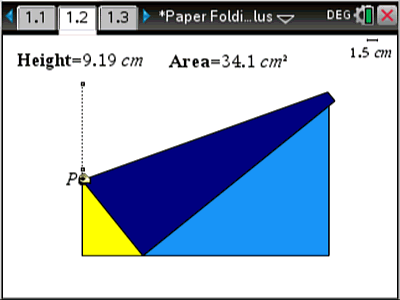# Activities

•• ##### AuthorAust Senior

20 Days

• ##### Device
•TI-Nspire™ CX CAS
• ##### Software

TI-Nspire™ CAS

4.4

• ##### Other Materials
A4 Paper and Ruler

## Paper Folding#### Activity Overview

In this activity students maximize the area of a triangle formed when a piece of A4 paper is folded in a special way. The activity provides an opportunity to explore the problem practically followed by an animated TI-Nspire file that automatically generates data so that students are able to check the validity of their data before proceeding. The unusual general result from this task makes the activity quite intriguing.

#### Objectives

• Create a function/relationship from a diagram
• Use calculus to find the derivative
• Determine maximum value from the derivative
• Generalise the solution

#### Vocabulary

• Derivative
• Maximum / Minimum

#### About the Lesson

Origami meets calculus! In this activity students fold an A4 piece of paper to form a triangle (as per the included instructions). Students determine the area of the triangle with a view to maximimising this quantity. Students can then use the dynamic representation in the TI-Nspire file to do the same, determine an algebraic formula for the area of the triangle and use calculus to find the maximum The result is surprisingly simple!